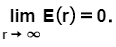# Problem: A solid ball of radius rb has a uniform charge density ρ.Part AWhat is the magnitude of the electric field E(r) at a distance r &gt; rb from the center of the ball? Express your answer in terms of ρ, rb, r, and ϵ0.Part BWhat is the magnitude of the electric field E(r) at a distance r &lt; rb from the center of the ball?Express your answer in terms of ρ, r, rb, and ϵ0.Part C.Let E(r) represent the electric field due to the charged ball throughout all of space. Which of the following statements about the electric field are true?1. E(0) = 02. E(rb) = 0.3. 4. The maximum electric field occurs when r = 0.5. The maximum electric field occurs when r = rb.6. The maximum electric field occurs as r → ∞.

###### FREE Expert Solution

Part A

The magnitude of the electric field can be calculated from the center of the ball.

According to Gauss's law:

$\mathbf{\int }\mathbit{E}\mathbf{·}\mathbit{d}\mathbit{A}\mathbf{=}\frac{\mathbf{q}}{{\mathbf{\epsilon }}_{\mathbf{0}}}$

Let's consider the ball to be a sphere.

89% (60 ratings)###### Problem Details

A solid ball of radius rb has a uniform charge density ρ.

Part A

What is the magnitude of the electric field E(r) at a distance r > rb from the center of the ball? Express your answer in terms of ρ, rb, r, and ϵ0.

Part B

What is the magnitude of the electric field E(r) at a distance < rb from the center of the ball?

Part C.

Let E(r) represent the electric field due to the charged ball throughout all of space. Which of the following statements about the electric field are true?

1. E(0) = 0

2. E(rb) = 0.

3.4. The maximum electric field occurs when r = 0.

5. The maximum electric field occurs when r = rb.

6. The maximum electric field occurs as r → ∞.